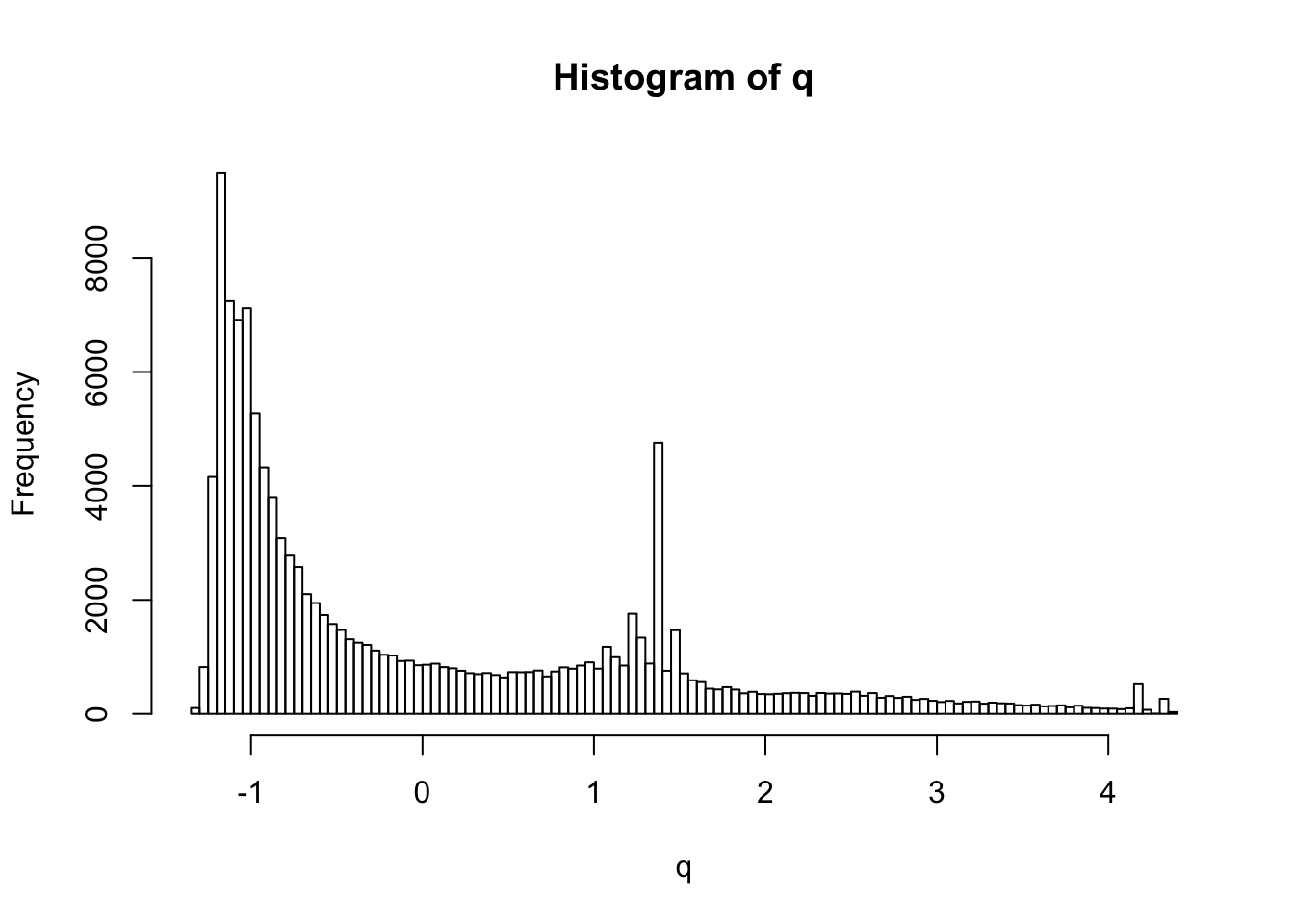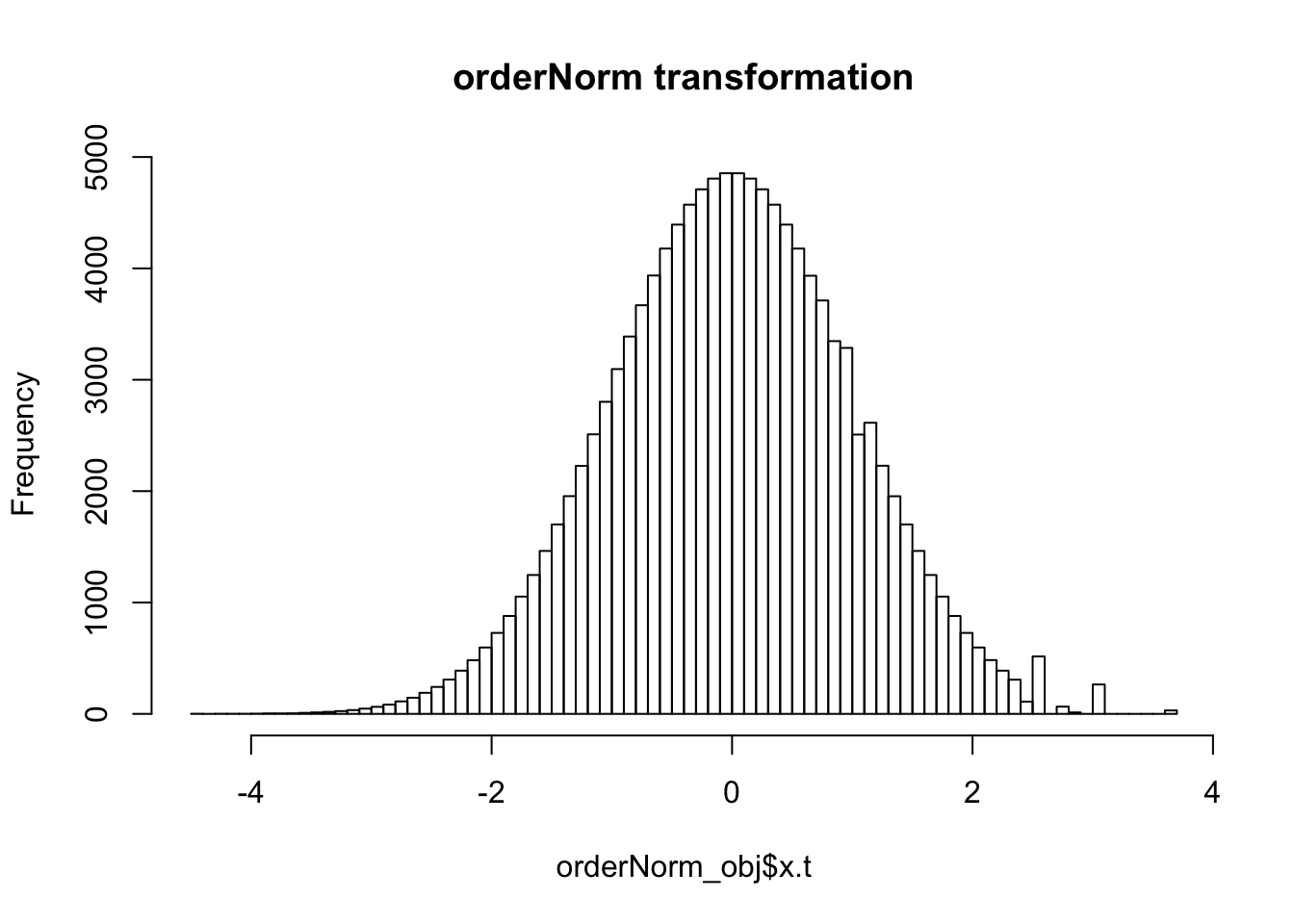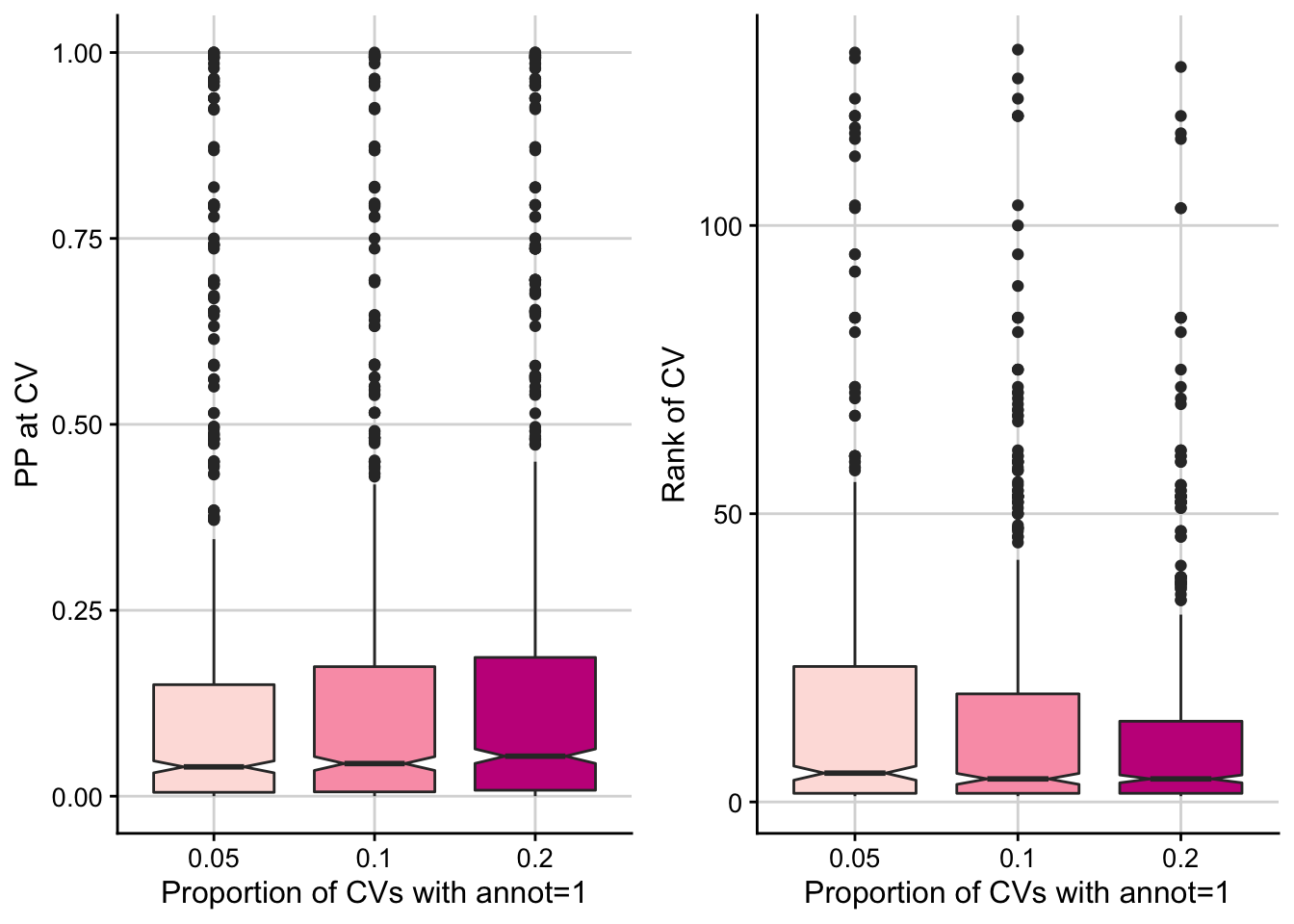## 1. cFDR

### Transform q values to normal distribution

A quick fix would be to convert the q values to a normal distribution and then directly use James’ functions.

The bestNormalize package (https://cran.r-project.org/web/packages/bestNormalize/vignettes/bestNormalize.html) allows comparison between different normalisation methods (where normalisation refers to making data normal, i.e. not just $$[0,1]$$).

mca_df <- readRDS("res.mca.mjca.RDS")
q <- mca_df[,1]
hist(q, breaks = 100)I use the package to find the recommended method for normalisation. The package suggests the ordered quantile (ORQ) normalization via orderNorm, a normalization technique based off of a rank mapping to the normal distribution, which guarantees normally distributed transformed data (if ties are not present).

library(bestNormalize)
(BNobject <- bestNormalize(q))
## Warning in bestNormalize(q): boxcox  did not work;  Error in estimate_boxcox_lambda(x, ...) : x must be positive
## Warning in orderNorm(standardize = TRUE, warn = TRUE, x = c(1.23253769877005, : Ties in data, Normal distribution not guaranteed
## Best Normalizing transformation with 121879 Observations
##  Estimated Normality Statistics (Pearson P / df, lower => more normal):
##  - No transform: 248.0488
##  - Log_b(x+a): 62.4443
##  - sqrt(x+a): 91.907
##  - exp(x): 2179.1578
##  - arcsinh(x): 291.9998
##  - Yeo-Johnson: 131.9365
##  - orderNorm: 4.6778
## Estimation method: Out-of-sample via CV with 10 folds and 5 repeats
##
## Based off these, bestNormalize chose:
## orderNorm Transformation with 121879 nonmissing obs and ties
##  - 102874 unique values
##  - Original quantiles:
##     0%    25%    50%    75%   100%
## -1.344 -1.038 -0.620  0.997  4.357

The annotation data is now normally distributed.

orderNorm_obj <- orderNorm(q)
## Warning in orderNorm(q): Ties in data, Normal distribution not guaranteed
hist(orderNorm_obj$x.t, main = "orderNorm transformation", breaks = 100)### Problem James’ code does not allow p or q $$<1e-300$$ which corresponds to 50% of the dataset. final_dataset <- readRDS("final_dataset.RDS") q <- readRDS("transformed_q.RDS") p <- final_dataset$p

source("cfdr_functions.R")

# p[which(p<1e-300)] <- 1e-300 # to avoid errors in James' code
# q[which(qs<1e-300)] <- 1e-300

candidate_indices <- which(p < 1)

chr <- final_dataset$chrom est_q0_pars <- fit.2g(q[which(p>0.5)])$pars

# prepare containers
fold <- vector(mode = "list", length = length(unique(chr)))
ind <- vector(mode = "list", length = length(unique(chr)))
L <- vector(mode = "list", length = length(unique(chr)))
v <- vector(mode = "list", length = length(unique(chr)))

for(i in 1:length(unique(chr))){
fold[[i]] <- which(chr == i)
ind[[i]] <- intersect(candidate_indices, fold[[i]])
L[[i]] <- vl(p, q, indices=ind[[i]], mode=2, fold=fold[[i]], gx = min(p[ind[[i]]]));  # compute L curves

v[[i]] <- il(L[[i]], pi0_null = est_q0_pars, sigma_null = est_q0_pars, distx="norm", gx = min(p[ind[[i]]])) # integrate over L curves
}

save(p, q, v, file = "transform_res.RData")

## 2. Figure for fine-mapping review

1. On the HPC (in review_PAINTORfig) generate many simulated locus files:
1. Fix OR=1.1, NN=10000 and randomly select a CV to generate Z scores for the “medium” LD region (714 SNPs).
2. Generate corresponding random annotation vector using rbinom(length(z), 1, pi) with pi=0.1.
3. Save locus file (consisting of Z scores), ld file (note that this will be the same for all simulations) and annotations file to be used as input into PAINTOR.
4. Store the iCV and binary annotaion at the CV (in review_PAINTORfig/res/misc/)
5. REPEAT (i)-(iv) many times (600 times).
2. Select subsets of datasets to be used as input into PAINTOR (see make_inputfiles.R):
1. Select 10 collections of 100 locus files whereby the proportion of CVs with annotation equal to 1 is pi (annotation uninformative). Make input.files and run PAINTOR for each of these 10. Save the PP at the CV in each (so have 10*100 PPs).
2. Select 10 collections of 100 locus files whereby the proportion of CVs with annotation equal to 1 is 0.2 (annotation informative - CVs more likely to have it). Make input.files and run PAINTOR for each of these 10. Save the PP at the CV in each (so have 10*100 PPs).
3. Select 10 collections of 100 locus files whereby the proportion of CVs with annotation equal to 1 is 0.05 (annotation informative - CVs less likely to have it). Make input.files and run PAINTOR for each of these 10. Save the PP at the CV in each (so have 10*100 PPs).
3. Compare PPs for CVs.

### Results

res_pi <- readRDS("/Users/anna/PAINTOR_V3.0/misc/res_pi.RDS")

res_pi <- res_pi[-which(res_pi$PPs==1 & res_pi$ranks!=1),]
res_lower <- res_lower[-which(res_lower$PPs==1 & res_lower$ranks!=1),]
res_higher <- res_higher[-which(res_higher$PPs==1 & res_higher$ranks!=1),]